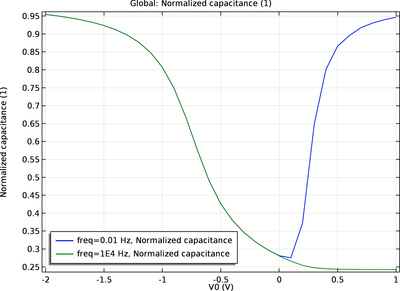# アプリケーションギャラリ

## MOSCAP 1D Small Signal

Application ID: 53531

The metal-silicon-oxide (MOS) structure is the fundamental building block for many silicon planar devices. Its capacitance measurements provide a wealth of insight into the working principles of such devices. This tutorial constructs a simple 1D model of a MOS capacitor (MOSCAP). Both the low- and high-frequency C-V curves are computed using the approach of small-signal analysis. The model employs the quasi-Fermi level formulation and the Semiconductor Equilibrium study step.This model example illustrates applications of this type that would nominally be built using the following products: